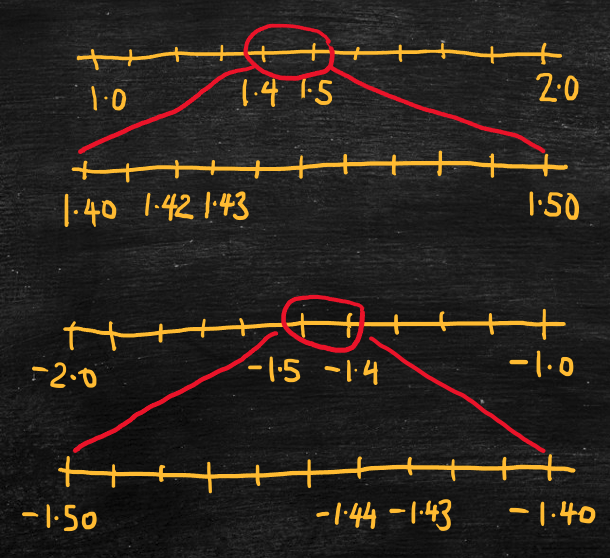Part 1: Numbers

# 1.1: Comparing numbers using a number line

Notes

A number line is a useful tool for representing decimal numbers, particularly when comparing numbers to determine which of two numbers is greater. Number lines should always be drawn from left to right (i.e., with the least number on the left and the greatest on the right). For example,

• A number line between 1 and 2 would have 1 on the left and 2 on the right.
• A number line between –2 and –1 would have –2 on the left and –1 on the right.
• A number line between –0.1 and 0.1 would have –0.1 on the left and 0.1 on the right.

Tick marks on the number line divide the distance between the numbers on the ends into equal-sized intervals. For example,

• A number line between 1 and 2 could be divided into tenths that mark off 1.0, 1.1, 1.2, …, 1.9, 2.0.
• A number line between –2 and –1 could be divided into tenths that mark off –2.0, –1.9, –1.8, …, –1.1, –1.0.
• A number line between –0.1 and 0.1 could be divided into hundredths that mark off –0.10, –0.09, –0.08, …, –0.01, 0, 0.01, …, 0.09, 0.10.

We can “zoom in” to intervals on the number line to represent decimal numbers with more decimal places. For example,

• On the number line between 1 and 2, zoom in to the interval between 1.4 and 1.5 to represent numbers like 1.42 and 1.43.
• On the number line between –2 and –1, zoom in to the interval between –1.5 and –1.4 to represent numbers like –1.44 and –1.43.
• On the number line between –0.1 and 0.1, zoom in to the interval between 0.04 and 0.05 to represent numbers like 0.041 and 0.042.

When comparing two decimal numbers, one number is greater than another if it is farther to the right on the number line. For example,

• 1.43 > 1.42 since 1.43 is farther to the right on the number line.
• –1.43 > –1.44 since –1.43 is farther to the right on the number line.
• 0.042 > 0.041 since 0.042 is farther to the right on the number line.Numbers lines between 1 and 2, 1.4 and 1.5, –2 and –1, and –1.5 and –1.4.

Equalize the length of a decimal number with zeros to help compare numbers with a different number of decimal places. For example,

• 1.42 > 1.4 since we can write 1.42 > 1.40.
• –1.4 > –1.43 since we can write –1.40 > –1.43.
• 0.14 > 0.056 since we can write 0.140 > 0.056.

The video below shows how to zoom in to number lines to compare decimal numbers.

Practice Exercises

Do the following exercises to practice ordering decimal numbers. Visualize the numbers on a number line to help you determine where to place the numbers in order from least on the left to greatest on the right.